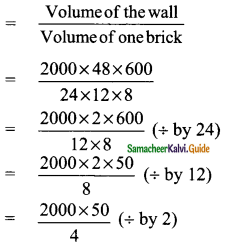Students can download Maths Chapter 7 Mensuration Ex 7.3 Questions and Answers, Notes, Samacheer Kalvi 9th Maths Guide Pdf helps you to revise the complete Tamilnadu State Board New Syllabus, helps students complete homework assignments and to score high marks in board exams.

## Tamilnadu Samacheer Kalvi 9th Maths Solutions Chapter 7 Mensuration Ex 7.3

Question 1.
Find the volume of a cuboid whose dimensions are
(i) length = 12 cm, breadth = 8 cm, height = 6 cm
(ii) length = 60 m, breadth = 25 m, height = 1.5 m
Solution:
(i) Here l = 12 cm, b = 8 cm, h = 6 cm
Volume of a cuboid = l × b × h
= (12 × 8 × 6) cm³
= 576 cm³

(ii) Here l = 60 m, b = 25 m. h = 1.5 m
Volume of a cuboid = l × b × h
= 60 × 25 × 1.5 m³
= 2250 m³Question 2.
The dimensions of a match box are 6 cm × 3.5 cm × 2.5 cm. Find the volume of a packet containing 12 such match boxes.
Solution:
Length of a match box (l) = 6 cm
Breadth of a match box (b) = 3.5 cm
Height of a match box (h) = 2.5 cm
Volume of one match box = l × b × h cu. units
= 6 × 3.5 × 2.5 cm³
= 52.5 cm³
Volume of 12 match box = 12 × 52.5 cm³
= 630 cm³Question 3.
The length, breadth and height of a chocolate box are in the ratio 5 : 4 : 3. If its volume is 7500 cm³, then find its dimensions.
Solution:
Let the length of a chocolate be 5x, the breadth of a chocolate be 4x, and the height of a chocolate be 3x.
Volume of a chocolate = 7500 cm³
l × b × h = 7500
5x × 4x × 3x = 7500
5 × 4 × 3 × x³ = 7500
x³ = $$\frac{7500}{5×4×3}$$
x³ = 125 ⇒ x³ = 5³
x = 5
∴ Length of a chocolate = 5 × 5 = 25 cm
Breath of a chocolate = 4 × 5 = 20 cm
Height of a chocolate = 3 × 5 = 15 cmQuestion 4.
The length, breadth and depth of a pond are 20.5 m, 16 m and 8 m respectively. Find the capacity of the pond in litres.
Solution:
Length of a pond (l) = 20.5 m
Breadth of a pond (b) = 16 m
Depth of a pond (h) = 8 m
Volume of the pond = l × b × h cu.units
= 20.5 × 16 × 8 m³
= 2624 m³ (1 cu. m = 1000 lit)
= (2624 × 1000) litres
= 2624000 litQuestion 5.
The dimensions of a brick are 24 cm × 12 cm × 8 cm. How many such bricks will be required to build a wall of 20 m length, 48 cm breadth and 6 m height?
Solution:
Length of a brick (l) = 24 cm
Breadth of a brick (b) = 12 cm
Depth of a brick (h) = 8 cm
Volume of a brick = lbh cu.units
Volume of one brick = 24 × 12 × 8 cm³
Length of a wall (l) = 20 m = 2000 cm
Breadth of a wall (b) = 48 cm
Height of a wall (h) = 6 m = 600 cm
Volume of a wall = l × b × h cu. units
= 2000 × 48 × 600 cm³
Number of bricks= 500 × 50 ( ÷ by 4)
= 25000 bricks
∴ Number of bricks = 25000Question 6.
The volume of a container is 1440 m³. The length and breadth of the container are 15 m and 8 m respectively. Find its height.
Solution:
Let the height of the container be “h”
Length of the container (l) = 15 m
Breadth of the container (b) = 8 m
Volume of the container = 1440 m³
l × b × h = 1440
15 × 8 × h = 1440
h = $$\frac{1440}{15×8}$$
= 12 m
∴ Height of the container = 12 mQuestion 7.
Find the volume of a cube each of whose side is
(i) 5 cm
(ii) 3.5 m
(iii) 21 cm
Solution:
(i) Side of a cube (a) = 5 cm
Volume of a cube = a³ cu. units
= 5 × 5 × 5 cm³
= 125 cm³

(ii) Side of a cube (a) = 3.5 m a³ cu. units
Volume of a cube = 3.5 × 3.5 × 3.5 m³
= 42.875 m³

(iii) Side of a cube (a) = 21 cm
Volume of a cube = a³ cu. units
= 21 × 21 × 21 cm³
= 9261 cm³Question 8.
A cubical milk tank can hold 125000 litres of milk. Find the length of its side in metres.
Solution:
Volume of the cubical tank = 125000 liters
= $$\frac{125}{1000}$$ m³ (1 cu.m = 1000 lit)
= 125 m³
a³ = 125 ⇒ a³ = 5³
a = 5
Side of a cube = 5 mQuestion 9.
A metallic cube with side 15 cm is melted and formed into a cuboid. If the length and height of the cuboid is 25 cm and 9 cm respectively then find the breadth of the cuboid.
Solution:
Side of a cube (a) = 15 cm
Length of a cuboid (l) = 25 cm
Height of a cuboid (h) = 9 cm
Volume of the cuboid = Volume of the cube
l × b × h = a³
25 × b × 9 = 15 × 15 × 15
b = $$\frac{15 × 15 × 15}{25 × 9}$$
= 15 cm
Breadth of the cuboid = 15 cm